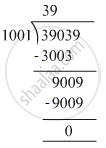# Divide and check your answer by the corresponding multiplication in the following: 39039 ​÷ 1001 - Mathematics

Fill in the Blanks

Divide and check your answer by the corresponding multiplication in the following:

39039 ​÷ 1001

#### SolutionDividend = 39039 , Divisor = 1001 , Quotient = 39 , Remainder = 0
Check : Divisor × Quotient + Remainder = 1001 × 39 + 0
= 39039
=Dividend
Hence, Dividend = Divisor × Quotient + Remainder

Is there an error in this question or solution?

#### APPEARS IN

RS Aggarwal Class 6 Mathematics
Chapter 3 Whole Numbers
Exercise 3E | Q 1.6 | Page 56